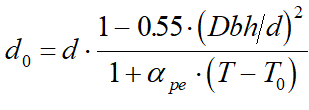# Calculation of Beta Ratio - Intergraph Smart Instrumentation - 13.1 - Reference - Hexagon PPM

## Integraph Smart Instrumentation Sizing Equations

Language
English (United States)
Product
Intergraph Smart Instrumentation
Search by Category
Reference
Smart Instrumentation Version
13.1
1. Calculate internal pipe diameter at flow temperature: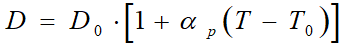2. Calculate correction factor for steam quality (for liquid, water, and steam, Fs=1):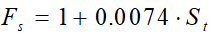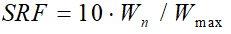4. Calculate differential pressure at normal flow rate: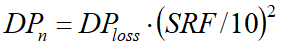5. Calculate pipe Reynolds number at normal flow rate: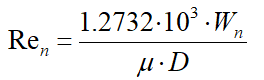6. Checking for critical flow.

1. Liquid/Water case:

1. If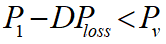then the flow is critical.

2. Gas/Steam case:

1. For Venturi tubes: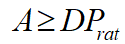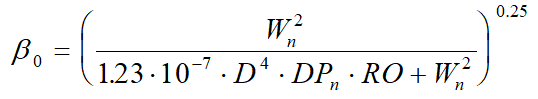2. For other restriction devices: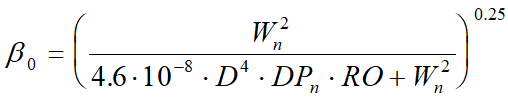3. Calculate critical pressure ratio and define whether the flow is crtiical: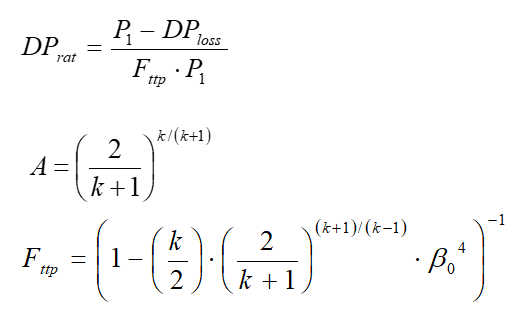Ifthen the flow is critical. If not, the flow is sub-critical.

7. Calculate b - ratio.

Case A Liquid/Water - critical flow

1. Calculate DP.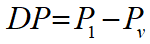2. Calculate first estimate for b - ratio.

For Venturi tubes: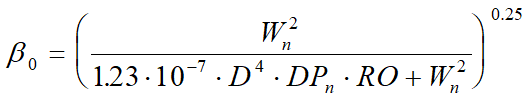For other restriction devices: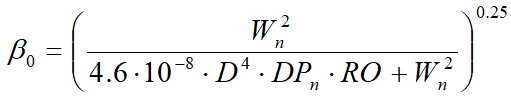3. Eps=1.

4. Calculate discharge coefficient. See the Appendix.

5. Calculate the new b - ratio.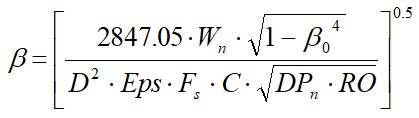6. Calculate the relative change in b - ratio.

7.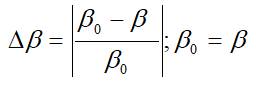8. Repeat steps d to f until the relative change in b - ratio is less than 0.0001.

Case B Gas/Steam - critical flow

1. Calculate gas expansion factor: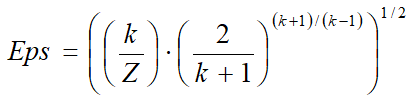2. Calculate discharge coefficient. See the Appendix.

3. Calculate first estimate for b -ratio (Wn[1b/h], T[r], P1{psi-a}, D[in]: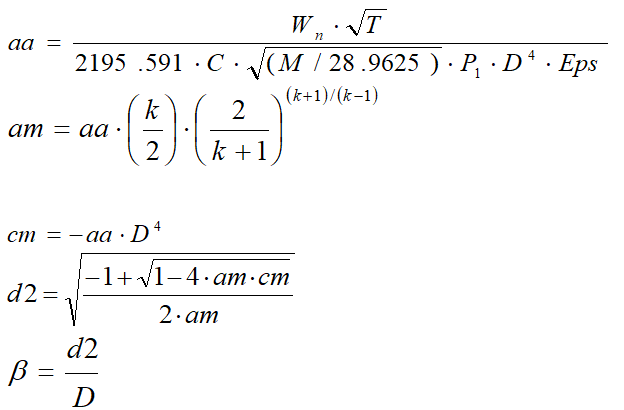4. Calculate orifice diameter at flow temperature: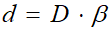5. Calculate bore Reynolds number at normal flow rate: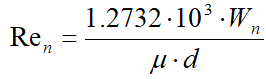6. Calculate discharge coefficient. See the Appendix.

7. Calculate new b -ration in accordance with equations in step c.

Case C Sub Critical flow

1. First estimate for DPn: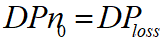2. Calculate first estimate for b - ratio.

1. For Venturi tubes: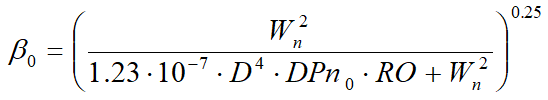2. For other restriction devices: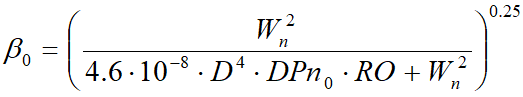3. Calculate gas expansion factor (for liquids/water, Eps = 1)

1. For Venturi tubes and nozzles: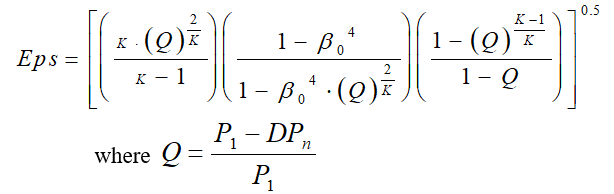2. For orifice plates: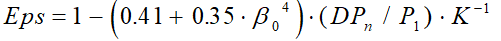4. Calculate discharge coefficient. See the Appendix.

5. Calculate new b - ratio: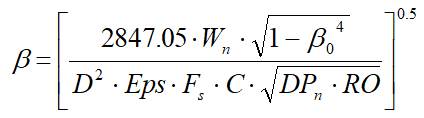6. Calculate new DP.

1. For classical Venturi tube: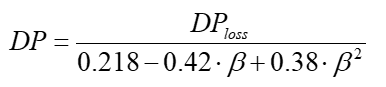2. For Nozzles: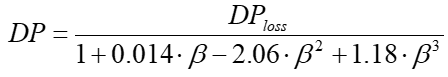3. For orifice plates: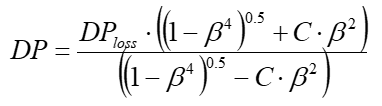7. Calculate new DPn: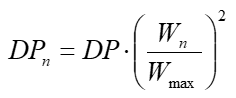8. Calculate the relative change in DPn: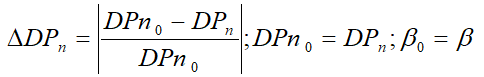9. In Case C, repeat steps c to h until the relative change in the DPn is less than 0.0001.

8. Calculate orifice diameter at flow temperature: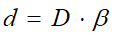9. Calculate orifice diameter at ambient temperature.

1. For Venturi tubes and nozzles: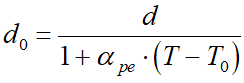2. For orifice plates: